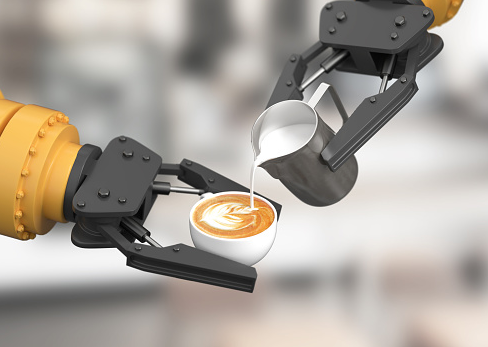## A Robotic Arm

Imagine a 40 cm long robotic arm that rotates around to serve you a coffee. If it weighs 80 N, what is the mass moment of inertia?Hint
Because the arm rotates/gyrates around, we can use the radius of gyration formula:
$$r_{m}=\sqrt{\frac{I}{m}}$$$where $$r$$ is the distance from a reference axis, $$I$$ is the mass moment of inertia, and $$m$$ is the mass. Hint 2 $$Force=mass \times acceleration$$$
$$Force=mass \times acceleration$$$Solving for mass: $$m=\frac{F}{a}=\frac{80N}{9.8m/s^2}=8.16\:kg$$$
Because the arm rotates/gyrates around, we can use the radius of gyration formula:
$$r_{m}=\sqrt{\frac{I}{m}}\rightarrow I=m\cdot r^{2}$$$where $$r$$ is the distance from a reference axis, $$I$$ is the mass moment of inertia, and $$m$$ is the mass. Thus, $$I=8.16kg\cdot (40cm)^2=13,061 \:kg\cdot cm^2$$$
$$13,061 \:kg\cdot cm^2$$\$